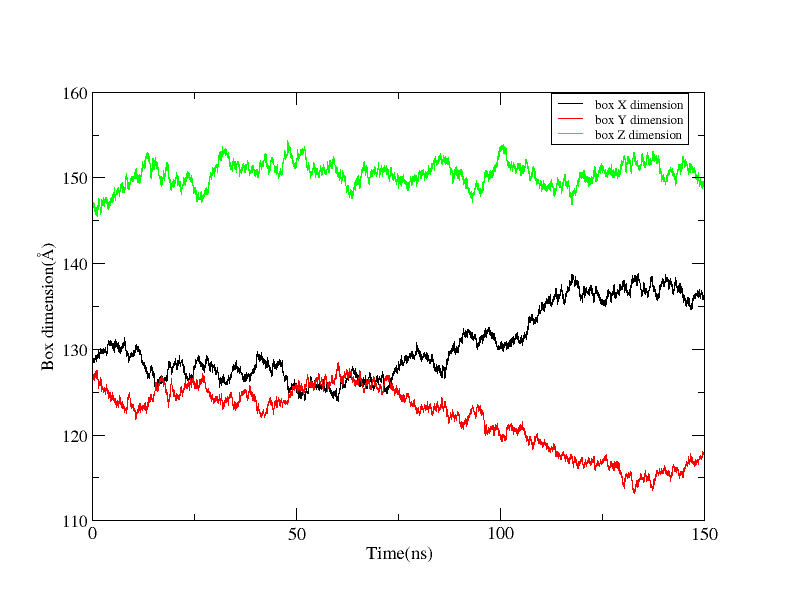# [AMBER] A confusion about the binding energy calculated by pbsa with implicit membrane model

From: 高振梅 <zmgao1019.163.com>
Date: Fri, 14 Sep 2018 21:25:56 +0800 (CST)

Dear AMBER,

I simulate large system (~240 thousand atoms) of membrane protein dimer embedded inside a POPC membrane. The system has ran 150ns completed through three consecutive runs. But the dimension of the periodic box in the X,Y direction changes too much.

the command in "prod.in" is:
50ns
&cntrl
imin=0, ntx=5, irest=1,
ntc=2, ntf=2, tol=0.0000001,
nstlim=25000000, ntt=3, gamma_ln=2.0,
temp0=303.0,
iwrap=1,
ntpr=5000, ntwr=5000, ntwx=5000,
dt=0.002, ig=-1,
ntb=2,ntp=2,cut=10.0,ioutfm=1,
/
Is this normal? Or is there any problem with the pressure of my system?